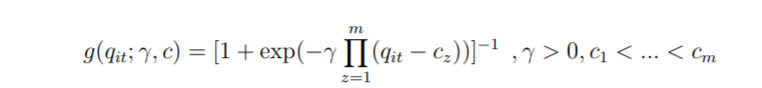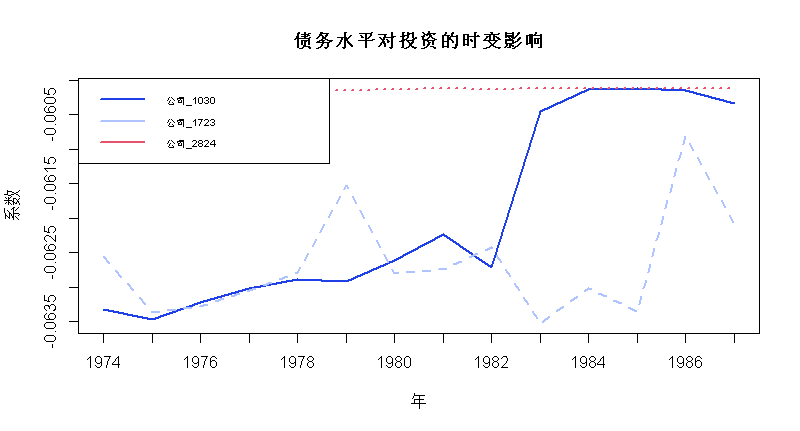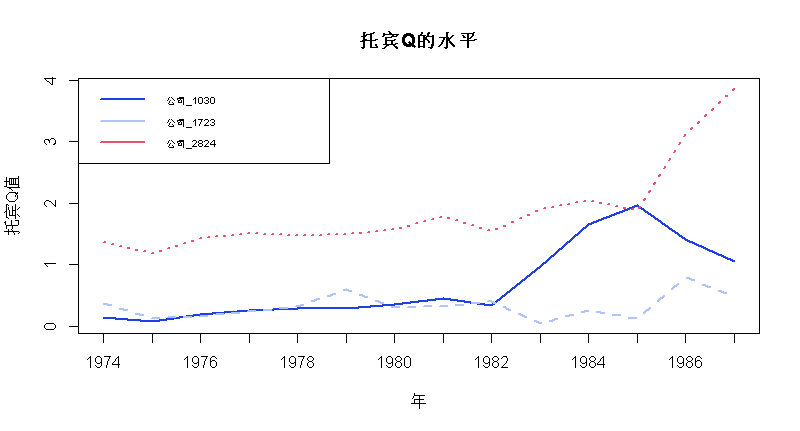# R语言时变面板平滑转换回归模型TV-PSTR分析债务水平对投资的影响

## 最近我们被客户要求撰写关于面板平滑转换回归(PSTR)的研究报告。建模过程包括三个阶段：表述，估计和评估。PSTR(data, dep='inva', indep=4:20, indep_k=c('vala','debta','cfa','sales'),tvars=c('vala'), iT=14) 

for (i in 1:n){
va_i<-vala[cusip==id[i]]
g<-(1+exp(-gamma*(va_i-c)))^(-1)
tvc_i<-est + mbeta*g 

matplot(tvc, type = 'l', lwd=2,col = 1:3, xaxt='n'
axis(1, at=1:nrow(tvc), labels=c(1974:1987)); legend("topleft", legend =
matplot(vala, type = 'l', lwd=2,col = 1:3, xaxt = 'n', xlab='年'
; axis(1, at=1:nrow(tvc), labels=c(1974:1987));
legend("topleft", legend = paste('公司',colnames(vala),sep=''), 

# 面板平滑转换回归(PSTR)分析案例实现### 随时关注您喜欢的主题

.这意味着投资弹性w.r.t托宾的Q需要用不同的方法来计算。

Kaizong Ye拓端研究室（TRL）的研究员。

​非常感谢您阅读本文，如需帮助请联系我们！QQ在线咨询

15121130882

0571-63341498

## 关注有关新文章的微信公众号

This will close in 0 seconds